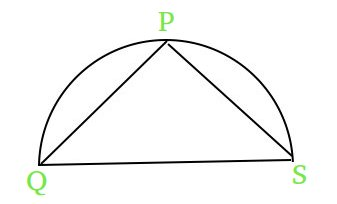# Maximize a value for a semicircle of given radius

We are given a semi circle with radius R. We can take any point on the circumference let it be P.Now, from that point P draw two lines to the two sides of diameter. Let the lines be PQ and PS.

The task is to find the maximum value of expression PS2 + PQ for a given R.Examples :

```Input : R = 1
Output : 4.25
(4*1^2 + 0.25) = 4.25

Input : R = 2
Output : 16.25
(4 * 2^2 + 0.25)= 16.25
```

## Recommended: Please try your approach on {IDE} first, before moving on to the solution.

Let F = PS^2 + PQ. We know QS = 2R (Diameter of the semicircle)
-> We also know that triangle PQS will always be right angled triangle irrespective of the position of point P on the circumference of circle

```1.)QS^2 = PQ^2 + PS^2 (Pythagorean Theorem)

2.) Adding and Subtracting PQ on the RHS
QS^2 = PQ^2 + PS^2 + PQ - PQ

3.) Since QS = 2R
4*R^2 + PQ - PQ^2 = PS^2 + PQ
=> 4*R^2 + PQ - PQ^2 = F

4.) Using the concept of maxima and minima
differentiating F with respect to PQ and equating
it to 0 to get the point of maxima for F i.e.
1 - 2 * PQ = 0
=> PQ = 0.5

5.) Now F will be maximum at F = 4*R^2 + 0.25
```

## C++

 `// C++ program to find  ` `// the maximum value of F ` `#include ` `using` `namespace` `std; ` ` `  `// Function to find the  ` `// maximum value of F ` `double` `maximumValueOfF (``int` `R) ` `{ ` `    ``// using the formula derived for  ` `    ``// getting the maximum value of F ` `    ``return` `4 * R * R + 0.25;      ` `} ` `     `  `// Drivers code ` `int` `main()  ` `{ ` `    ``int` `R = 3;  ` `    ``printf``(``"%.2f"``, maximumValueOfF(R));  ` `    ``return` `0; ` `} `

## JAVA

 `// JAVA program to find  ` `// the maximum value of F ` `import` `java.io.*; ` ` `  `class` `GFG ` `{ ` `     `  `    ``// Function to find the  ` `    ``// maximum value of F ` `    ``static` `double` `maximumValueOfF (``int` `R) ` `    ``{ ` `         `  `        ``// using the formula derived for  ` `        ``// getting the maximum value of F ` `        ``return` `4` `* R * R + ``0.25``;      ` `    ``} ` `     `  `    ``// Driver code ` `    ``public` `static` `void` `main (String[] args) ` `    ``{ ` `        ``int` `R = ``3``;  ` `        ``System.out.println(maximumValueOfF(R));  ` `    ``} ` `         `  `} ` ` `  `// This code is contributed  ` `// by anuj_67. `

## Python3

 `# python program to find  ` `# the maximum value of F ` ` `  `# Function to find the  ` `# maximum value of F ` `def` `maximumValueOfF (R): ` `     `  `    ``# using the formula derived for  ` `    ``# getting the maximum value of F ` `    ``return` `4` `*` `R ``*` `R ``+` `0.25` ` `  `      `  `# Drivers code ` `R ``=` `3` `print``(maximumValueOfF(R)) ` ` `  `# This code is contributed by Sam007. `

## C#

 `// C# program to find the  ` `// maximum value of F ` `using` `System; ` ` `  `class` `GFG  ` `{ ` `     `  `    ``// Function to find the  ` `    ``// maximum value of F ` `    ``static` `double` `maximumValueOfF (``int` `R) ` `    ``{ ` `         `  `        ``// using the formula derived for  ` `        ``// getting the maximum value of F ` `        ``return` `4 * R * R + 0.25;      ` `    ``} ` `     `  `    ``// Driver code ` `    ``public` `static` `void` `Main () ` `    ``{ ` `        ``int` `R = 3;  ` `        ``Console.WriteLine(maximumValueOfF(R));  ` `    ``} ` `         `  `} ` ` `  `// This code is contributed by Sam007. `

## PHP

 ` `

Output :

```36.25
```

My Personal Notes arrow_drop_upCheck out this Author's contributed articles.

If you like GeeksforGeeks and would like to contribute, you can also write an article using contribute.geeksforgeeks.org or mail your article to contribute@geeksforgeeks.org. See your article appearing on the GeeksforGeeks main page and help other Geeks.

Please Improve this article if you find anything incorrect by clicking on the "Improve Article" button below.

Improved By : vt_m, Sam007

Article Tags :
Practice Tags :

Be the First to upvote.

Please write to us at contribute@geeksforgeeks.org to report any issue with the above content.